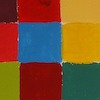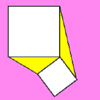# Resources tagged with: Area - squares and rectangles

Filter by: Content type:
Age range:
Challenge level:

### There are 8 results

Broad Topics > Measuring and calculating with units > Area - squares and rectangles### Pythagoras for a Tetrahedron

##### Age 16 to 18 Challenge Level:

In a right-angled tetrahedron prove that the sum of the squares of the areas of the 3 faces in mutually perpendicular planes equals the square of the area of the sloping face. A generalisation. . . .### Compare Areas

##### Age 14 to 16 Challenge Level:

Which has the greatest area, a circle or a square, inscribed in an isosceles right angle triangle?### Semi-detached

##### Age 14 to 16 Challenge Level:

A square of area 40 square cms is inscribed in a semicircle. Find the area of the square that could be inscribed in a circle of the same radius.### Take a Square

##### Age 14 to 16 Challenge Level:

Cut off three right angled isosceles triangles to produce a pentagon. With two lines, cut the pentagon into three parts which can be rearranged into another square.### The Square Under the Hypotenuse

##### Age 14 to 16 Challenge Level:

Can you work out the side length of a square that just touches the hypotenuse of a right angled triangle?### Proof of Pick's Theorem

##### Age 16 to 18 Challenge Level:

Follow the hints and prove Pick's Theorem.### Trig Rules OK

##### Age 16 to 18 Challenge Level:

Change the squares in this diagram and spot the property that stays the same for the triangles. Explain...### Warmsnug Double Glazing

##### Age 14 to 16 Challenge Level:

How have "Warmsnug" arrived at the prices shown on their windows? Which window has been given an incorrect price?